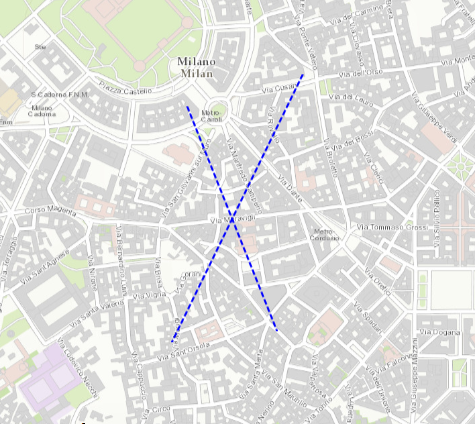# Using GeometryEngine. Intersection computes cannot get the Intersection of two lines

885
2
03-13-2017 06:21 PMby
New Contributor III

Using two lines intersect in the MapView, get the line, is not the point, why? And this line is not shown on the mapMy code is as follows：

1 Solution

Accepted SolutionsbyEsri Contributor

The `GeometryEngine.Intersection` method only returns geometries of the same dimension as the input geometries. So for two polylines it will only return a polyline not a point. In the case here where the lines intersect at a single point it will return an empty polyline.

This isn't helpful for finding the intersection point of two crossing lines. The next update of the Runtime API will have another `GeometryEngine` method that returns multiple geometries including geometries of lesser dimensions. You'll be able to use this to get the intersection point of two polylines.

In the meantime, you may be able to use another `GeometryEngine` method to help find the intersection points. For instance, `GeometryEngine.Cut` with the same input polylines will return separate lines and you can match up the start and end points to infer the intersection point. Not ideal, but would work for the simple case.

2 RepliesbyEsri Contributor

The `GeometryEngine.Intersection` method only returns geometries of the same dimension as the input geometries. So for two polylines it will only return a polyline not a point. In the case here where the lines intersect at a single point it will return an empty polyline.

This isn't helpful for finding the intersection point of two crossing lines. The next update of the Runtime API will have another `GeometryEngine` method that returns multiple geometries including geometries of lesser dimensions. You'll be able to use this to get the intersection point of two polylines.

In the meantime, you may be able to use another `GeometryEngine` method to help find the intersection points. For instance, `GeometryEngine.Cut` with the same input polylines will return separate lines and you can match up the start and end points to infer the intersection point. Not ideal, but would work for the simple case.by
New Contributor III